# Neural Networks from Scratch with Python Code and Math in Detail— I

## Building neural networks from scratch. From the math behind them to step-by-step implementation coding samples in Python with Google Colab

Author(s): Pratik Shukla, Roberto Iriondo

Last updated, June 29, 2020

Note: In our second tutorial on neural networks, we dive in-depth on the limitations and advantages of using neural networks. We show how to implement neural nets with hidden layers and how these lead to a higher accuracy rate on our predictions, along with implementation samples in Python on Google Colab.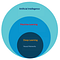Figure 1: Where neural networks fit in AI, machine learning, and deep learning.

# What is a neural network?

Neural networks form the base of deep learning, which is a subfield of machine learning, where the structure of the human brain inspires the algorithms. Neural networks take input data, train themselves to recognize patterns found in the data, and then predict the output for a new set of similar data. Therefore, a neural network can be thought of as the functional unit of deep learning, which mimics the behavior of the human brain to solve complex data-driven problems.

The first thing that comes to our mind when we think of “neural networks” is biology, and indeed, neural nets are inspired by our brains.

Let’s try to understand them: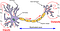Figure 2: An image representing a biological neuron | Source: Wikipedia 

In machine learning, the neurons’ dendrites refer to as input, and the nucleus process the data and forward the calculated output through the axon. In a biological neural network, the width (thickness) of dendrites defines the weight associated with it.

# 1. What is an Artificial Neural Network?

Simply put, an ANN represents interconnected input and output units in which each connection has an associated weight. During the learning phase, the network learns by adjusting these weights in order to be able to predict the correct class for input data.

For instance:

We encounter ourselves in a deep sleep state, and suddenly our environment starts to tremble. Immediately afterward, our brain recognizes that it is an earthquake. At once, we think of what is most valuable to us:

• Our beloved ones.
• Essential documents.
• Jewelry.
• Laptop.
• A pencil.

Now we only have a few minutes to get out of the house, and we can only save a few things. What will our priorities be in this case?

Perhaps, we are going to save our beloved ones first, and then if time permits, we can think of other things. What we did here is, we assigned a weight to our valuables. Each of the valuables at that moment is an input, and the priorities are the weights we assigned it to it.

The same is the case with neural networks. We assign weights to different values and predict the output from them. However, in this case, we do not know the associated weight with each input, so we make an algorithm that will calculate the weights associated with them by processing lots of input data.

# 2. Applications of Artificial Neural Networks:

## a. Classification of data:

Based on a set of data, our trained neural network predicts whether it is a dog or a cat?

## b. Anomaly detection:

Given the details about transactions of a person, it can say that whether the transaction is fraud or not.

## c. Speech recognition:

We can train our neural network to recognize speech patterns. Example: Siri, Alexa, Google assistant.

## d. Audio generation:

Given the inputs as audio files, it can generate new music based on various factors like genre, singer, and others.

## e. Time series analysis:

A well trained neural network can predict the stock price.

## f. Spell checking:

We can train a neural network that detects misspelled spellings and can also suggest a similar meaning for words. Example: Grammarly

## g. Character recognition:

A well trained neural network can detect handwritten characters.

## h. Machine translation:

We can develop a neural network that translates one language into another language.

## i. Image processing:

We can train a neural network to process an image and extract pieces of information from it.

# 3. General Structure of an Artificial Neural Network (ANN):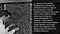Figure 5: The perceptron by Frank Rosenblatt | Source: Machine Learning Department at Carnegie Mellon University

# 4. What is a Perceptron?

A perceptron is a neural network without any hidden layer. A perceptron only has an input layer and an output layer.

## Where we can use perceptrons?

Perceptrons’ use lies in many case scenarios. While a perceptron is mostly used for simple decision making, these can also come together in larger computer programs to solve more complex problems.

For instance:

1. Give access if a person is a faculty member and deny access if a person is a student.
2. Provide entry for humans only.
3. Implementation of logic gates .

## Steps involved in the implementation of a neural network:

A neural network executes in 2 steps :

## 1. Feedforward:

On a feedforward neural network, we have a set of input features and some random weights. Notice that in this case, we are taking random weights that we will optimize using backward propagation.

## 2. Backpropagation:

During backpropagation, we calculate the error between predicted output and target output and then use an algorithm (gradient descent) to update the weight values.

## Why do we need backpropagation?

While designing a neural network, first, we need to train a model and assign specific weights to each of those inputs. That weight decides how vital is that feature for our prediction. The higher the weight, the greater the importance. However, initially, we do not know the specific weight required by those inputs. So what we do is, we assign some random weight to our inputs, and our model calculates the error in prediction. Thereafter, we update our weight values and rerun the code (backpropagation). After individual iterations, we can get lower error values and higher accuracy.

## Summarizing an Artificial Neural Network:

1. Take inputs
3. Assign random weights to input features
4. Run the code for training.
5. Find the error in prediction.
6. Update the weight by gradient descent algorithm.
7. Repeat the training phase with updated weights.
8. Make predictions.

# 5. Perceptron Example:

Below is a simple perceptron model with four inputs and one output.

What we have here is the input values and their corresponding target output values. So what we are going to do, is assign some weight to the inputs and then calculate their predicted output values.

In this example we are going to calculate the output by the following formula:

For the sake of this example, we are going to take the bias value = 0 for simplicity of calculation.

a. Let’s take W = 3 and check the predicted output.

b. After we have found the value of predicted output for W=3, we are going to compare it with our target output, and by doing that, we can find the error in the prediction model. Keep in mind that our goal is to achieve minimum error and maximum accuracy for our model.

c. Notice that in the above calculation, there is an error in 3 out of 4 predictions. So we have to change the parameter values of our weight to set in low. Now we have two options:

1. Increase weight
2. Decrease weight

First, we are going to increase the value of the weight and check whether it leads to a higher error rate or lower error rate. Here we increased the weight value by 1 and changed it to W = 4.

d. As we can see in the figure above, is that the error in prediction is increasing. So now we can conclude that increasing the weight value does not help us in reducing the error in prediction.

e. After we fail in increasing the weight value, we are going to decrease the value of weight for it. Furthermore, by doing that, we can see whether it helps or not.

f. Calculate the error in prediction. Here we can see that we have achieved the global minimum.

In figure 17, we can see that there is no error in prediction.

Now what we did here:

1. First, we have our input values and target output.
2. Then we initialized some random value to W, and then we proceed further.
3. Last, we calculated the error for in prediction for that weight value. Afterward, we updated the weight and predicted the output. After several trial and error epochs, we can reduce the error in prediction.

So, we are trying to get the value of weight such that the error becomes minimum. We need to figure out whether we need to increase or decrease the weight value. Once we know that, we keep on updating the weight value in that direction until error becomes minimum. We might reach a point where if further updates occur to the weight, the error will increase. At that time, we need to stop, and that is our final weight value.

In real-life data, the situation can be a bit more complex. In the example above, we saw that we could try different weight values and get the minimum error manually. However, in real-life data, weight values are often decimal (non-integer). Therefore, we are going to use a gradient descent algorithm with a low learning rate so that we can try different weight values and obtain the best predictions from our model.

# 6. Sigmoid Function:

A sigmoid function serves as an activation function in our neural network training. We generally use neural networks for classifications. In binary classification, we have 2 types. However, as we can see, our output value can be any possible number from the equation we used. To solve that problem, we use a sigmoid function. Now for classification, we want our output values to be 0 or 1. So to get values between 0 and 1 we use the sigmoid function. The sigmoid function converts our output values between 0 and 1.

Let’s have a look at it:

Let’s visualize our sigmoid function with Python:

Output:

Explanation:

In figure 21 and 22, for any input values, the value of the sigmoid function will always lie between 0 and 1. Here notice that for negative numbers, the output of the sigmoid function is ≤0.5, or we can say closer to zero, and for positive numbers, the output is going to be >0.5, or we can say closer to 1.

# 7. Neural Network Implementation from Scratch:

We are going to do is implement the “OR” logic gate using a perceptron. Keep in mind that here we are not going to use any of the hidden layers.

## What is logical OR Gate?

Straightforwardly, when one of the inputs is 1, the output of the OR gate is going to be 1. It means that the output is 0 only when both of the inputs are 0.

## Perceptron for the OR gate:

Next, we are going to assign some weights to each of the input values and calculate it.

## Example: (Calculating Manually)

a. Calculate the input for o1:

b. Calculate the output value:

Notice that from our truth table, we can see that we wanted the output of 1, but what we get here is 0.68997. Now we need to calculate the error and then backpropagate and then update the weight values.

c. Error Calculation:

Next, we are going to use Mean Squared Error for calculating the error :

The summation sign (Sigma symbol) means that we have to add our error for all our input sets. Here we are going to see how that works for only one input set.

We have to do the same for all the remaining inputs. Now that we have found the error, we have to update the values of weight to make the error minimum. For updating weight values, we are going to use a gradient descent algorithm.

# 8. What is Gradient Descent?

Gradient Descent is a machine learning algorithm that operates iteratively to find the optimal values for its parameters. It takes into account, user-defined learning rate, and initial parameter values.

Working: (Iterative)

2. Calculate cost.

3. Update values using the update function.

4. Returns minimized cost for our cost function

## Why do we need it?

Generally, what we do is, we find the formula that gives us the optimal values for our parameter. However, in this algorithm, it finds the value by itself!

Interesting, isn’t it?

We are going to update our weight with this algorithm. First of all, we need to find the derivative f(X).

# 9. Derivation of the formula used in a neural network

Next, what we want to find is how a particular weight value affects the error. To find that we are going to apply the chain rule.

Afterward, what we have to do is we have to find values for these three derivatives.

In the following images, we have tried to show the derivation of each of these derivatives to showcase the math behind gradient descent.

d. Calculating derivatives:

In our case:

Output = 0.68997
Target = 1

e. Finding the second part of the derivative:

To understand it step-by-step:

e.a. Value of outo1:

e.b. Finding the derivative with respect to ino1:

e.c. Simplifying it a bit to find the derivative easily:

e.d. Applying chain rule and power rule:

e.e. Applying sum rule: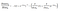Figure 41: Applying sum rule to outo1 with respect to ino1

e.f. The derivative of constant is zero:

e.g. Applying exponential rule and chain rule:

e.h. Simplifying it a bit:

e.i. Multiplying both negative signs:

e.j. Put the negative power in the denominator:

That is it. However, we need to simplify it as it is a little complex for our machine learning algorithm to process for a large number of inputs.

e.k. Simplifying it:

e.l. Further simplification:

e.l. Separate the parts:

e.m. Simplify:

e.n. Now we all know the value of outo1 from equation 1:

e.o. From that we can derive the following final derivative:

e.p. Calculating the value of our input:

f. Finding the third part of the derivative :

f.a Value of `ino`:

f.b. Finding derivative:

All the other values except w2 will be considered constant here.

f.c Calculating both values for our input:

f.d. Putting it all together:

f.e. Putting it in our main equation:

f.f. We can calculate:

Notice that the value of the weight has increased here. We can calculate all the values in this way, but as we can see, it is going to be a lengthy process. So now we are going to implement all the steps in Python.

## Summary of The Manual Implementation of a Neural Network: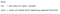Figure 61: Remember ino = input value * weight | outo = output value after applying the sigmoid function

a. Input for perceptron:

b. Applying sigmoid function for predicted output :

c. Calculate the error:

d. Changing the weight value based on gradient descent formula:

e. Calculating the derivative:

f. Individual derivatives:

Source: Image created by the author.

Source: Image created by the author.

g. After then we run the same code with updated weight values.

Let’s code:

# 10. Implementation of a Neural Network In Python:

## 10.1 Import Required libraries:

First, we are going to import Python libraries. We are using NumPy for the calculations:

Next, we are going to take input values for which we want to train our neural network. Here we can see that we have taken two input features. In actual data sets, the value of the input features is mostly high.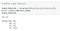Figure 71: Assign the input values to train our neural network

## 10.3 Target Output:

For the input features, we want to have a specific output for specific input features. It is called the target output. We are going to train the model that gives us the target output for our input features.

## 10.3 Assign the Weights :

Next, we are going to assign random weights to the input features. Note that our model is going to modify these weight values to be optimum. At this point, we are taking these values randomly. Here we have two input features, so we are going to take two weight values.

## 10.4 Adding Bias Values and Assigning a Learning Rate :

Now here we are going to add the bias value. The value of bias = 1. However, the weight assigned to it is random at first, and our model will optimize it for our target output.

The other parameter is called the learning rate(LR). We are going to use the learning rate in a gradient descent algorithm to update the weight values. Generally, we keep the learning rate as low as possible so that we can achieve a minimum error rate.

## 10.5 Applying a Sigmoid Function:

Once we have our weight values and input features, we are going to send it to the main function that predicts the output. Now notice that our input features and weight values can be anything, but here we want to classify data, so we need the output between 0 and 1. For such, we are going to a sigmoid function.

## 10.6 Derivative of sigmoid function:

In gradient descent algorithm we are going to need the derivative of the sigmoid function.

## 10.7 The main logic for predicting output and updating the weight values:

We are going to explain the following code step-by-step.

## How does it work?

First of all, the code above will need to run approximately 10,000 times. Keep in mind that if we only run this code a few times, then probably we are going to have a higher error rate. Therefore, in short, we can say that we are going to update the weight values 10,000 times to reach the optimal value possible.

Next, what we need to do is multiply the input features with it is corresponding weight values, the values we are going to feed to the perceptron can be represented in the form of a matrix.

`in_o` represents the dot product of `input_features` and `weight`. Notice that the first matrix (input features) is of size (4*2), and the second matrix (weights) is of size (2*1). After multiplication, the resultant matrix is of size (4*1).

In the above representation, each of those boxes represents a value.

Now in our formula, we also have the bias value. Let’s understand it with simple matrix representation.

Next, we are going to add the bias value. Addition operation in the matrix is easy to understand. Such is the input for the sigmoid function. Afterward, we are going to apply the sigmoid function to our input value, which will give us the predicted output value between 0 and 1.

Next, we have to calculate the error in prediction. We generally use Mean Squared Error (MSE) for this, but here we are just going to use simple error function for simplicity in the calculation. Last, we are going to add the error for all of our four inputs.

Our ultimate goal is to minimize the error. To minimize the error, we can update the value of our weights. To update the weight value, we are going to use a gradient descent algorithm.

To find the derivative, we are going to need the values of some derivatives for our gradient descent algorithm. As we have already discussed, we are going to find 3 individual values for derivatives and then multiply it.

The first derivative is:

The second derivative is:

The third derivative is:

Notice that we can easily find the values of the first two derivatives as they are not dependent on inputs. Next, we store the values of the multiplication of the first two derivatives in the `deriv` variable. Now the values of these derivatives must be of the same size as the size of weights. The size of the weights is (2*1).

To find the final derivative, we need to find the transpose of our `input_features `and then we are going to multiply it with our `deriv` variable that is basically the multiplication of the other two derivatives.

Let’s have a look at the matrix representation of the operation.

On figure 83, the first matrix is the transposed matrix of `input_features`. The second matrix stores the values of the multiplication of the other two derivatives. Now see that we have stored these values in a matrix called `deriv_final`. Notice that, the size of `deriv_final` is (2*1) which is the same as the size of our weight matrix (2*1).

Afterward, we update the weight value, notice that we have all the values needed for updating our weight. We are going to use the following formula to update the weight values.

Last, we need to update the bias value. If we remember the diagram, we might have noticed that the value of bias weight is not dependent on the input. So we have to update it separately. In this case, we need the `deriv` values, as it is not dependent on the input values. To update the bias value, we go through the for loop for updating value at each input on every iteration.

## 10.8 Check the Values of Weight and Bias: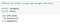Figure 85: Notice how our weights and values changed from the randomly assigned values

On figure 85, notice that our weight and bias values have changed from our randomly assigned values.

10.9 Predicting values :

Since we have trained our model, we can start to make predictions from it.

10.9.1 Prediction for (1,0):

Target value = 1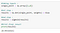Figure 86: Predicted output approximating 1 for target value = 1

On figure 86, we can see the predicted output is very near to 1.

10.9.2 Prediction for (1,1):

Target output = 1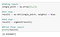Figure 87: Predicted value approximating 1 for target output = 1

On figure 87, we can see that the predicted output is very close to 1.

10.9.3 Prediction for (0,0):

Target output = 0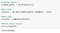Figure 88: Predicted value approximating 0 for target output = 0

On figure 88, we can see that the predicted output is very close to 0.

# Putting it all together:

`# Import required libraries:import numpy as np# Define input features:input_features = np.array([[0,0],[0,1],[1,0],[1,1]])print (input_features.shape)print (input_features)# Define target output:target_output = np.array([[0,1,1,1]])# Reshaping our target output into vector:target_output = target_output.reshape(4,1)print(target_output.shape)print (target_output)# Define weights:weights = np.array([[0.1],[0.2]])print(weights.shape)print (weights)# Bias weight:bias = 0.3# Learning Rate:lr = 0.05# Sigmoid function:def sigmoid(x):return 1/(1+np.exp(-x))# Derivative of sigmoid function:def sigmoid_der(x):return sigmoid(x)*(1-sigmoid(x))# Main logic for neural network:# Running our code 10000 times:for epoch in range(10000):inputs = input_features#Feedforward input:in_o = np.dot(inputs, weights) + bias #Feedforward output:out_o = sigmoid(in_o) #Backpropogation#Calculating errorerror = out_o - target_output#Going with the formula:x = error.sum()print(x)#Calculating derivative:derror_douto = errordouto_dino = sigmoid_der(out_o)#Multiplying individual derivatives:deriv = derror_douto * douto_dino #Multiplying with the 3rd individual derivative:#Finding the transpose of input_features:inputs = input_features.Tderiv_final = np.dot(inputs,deriv)#Updating the weights values:weights -= lr * deriv_final #Updating the bias weight value:for i in deriv: bias -= lr * i #Check the final values for weight and biasprint (weights)print (bias) #Taking inputs:single_point = np.array([1,0]) #1st step:result1 = np.dot(single_point, weights) + bias #2nd step:result2 = sigmoid(result1) #Print final resultprint(result2) #Taking inputs:single_point = np.array([1,1]) #1st step:result1 = np.dot(single_point, weights) + bias #2nd step:result2 = sigmoid(result1) #Print final resultprint(result2) #Taking inputs:single_point = np.array([0,0]) #1st step:result1 = np.dot(single_point, weights) + bias #2nd step:result2 = sigmoid(result1) #Print final resultprint(result2)`

# Why do we add bias?

Suppose if we have input values (0,0), the sum of the products of the input nodes and weights is always going to be zero. In this case, the output will always be zero, no matter how much we train our model. To resolve this issue and make reliable predictions, we use the bias term. In short, we can say that the bias term is necessary to make a robust neural network.

Therefore, how does the value of bias affects the shape of our sigmoid function? Let’s visualize it with some examples.

To change the steepness of the sigmoid curve, we can adjust the weight accordingly.

For instance: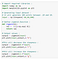Figure 89: Python code resembling our implementation of a neural net without bias values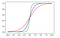Figure 90: Data visualization of our neural net

From the output, we can quickly notice that for negative values, the output of the sigmoid function is going to be ≤0.5. Moreover, for positive values, the output is going to be >0.5.

From the figure (red curve), you can see that if we decrease the value of the weight, it decreases the value of steepness, and if we increase the value of weight (green curve), it increases the value of steepness. However, for all of the three curves, if the input is negative, the output is always going to be ≤0.5. For positive numbers, the output is always going to be >0.5.

## What if we want to change this pattern?

For such case scenarios, we use bias values.

From the output, we can notice that we can shift the curves on the x-axis that helps us to change the pattern we show in the previous example.

## Summary: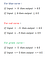Figure 93: Summary of our thresholds on shift curves for pattern changes

In neural networks:

• We can view bias as a threshold value for activation.
• Bias increases the flexibility of the model
• The bias value allows us to shift the activation function to the right or left.
• The bias value is most useful when we have all zeros (0,0) as input.

Let’s try to understand it with the same example we saw earlier. Nevertheless, here we are not going to add the bias value. After the model has trained, we will try to predict the value of (0,0). Ideally, it should be close to zero. Now let’s check out the following example.

# An Implementation Without Bias Value:

## c. Target output: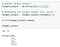Figure 96: Defining our target outputs, and reshaping our target output into a vector

## h. The main logic for training our model:

Here notice that we are not going to use bias values anywhere.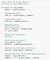Figure 101: Notice that we won’t be using bias on our neural net implementation

## i. Making predictions:

i.a. Prediction for (1,0) :

Target output = 1

From the predicted output we can see that it’s close to 1.

i.b. Prediction for (0,0) :

Target output = 0

Here we can see that it’s nowhere near 0. So we can say that our model failed to predict it. This is the reason for adding the bias value.

i.c. Prediction for (1,1):

Target output = 1

We can see that it’s close to 1.

# Putting it all together:

`# Import required libraries :import numpy as np# Define input features :input_features = np.array([[0,0],[0,1],[1,0],[1,1]])print (input_features.shape)print (input_features)# Define target output :target_output = np.array([[0,1,1,1]])# Reshaping our target output into vector :target_output = target_output.reshape(4,1)print(target_output.shape)print (target_output)# Define weights :weights = np.array([[0.1],[0.2]])print(weights.shape)print (weights)# Define learning rate :lr = 0.05# Sigmoid function :def sigmoid(x): return 1/(1+np.exp(-x))# Derivative of sigmoid function :def sigmoid_der(x): return sigmoid(x)*(1-sigmoid(x))# Main logic for neural network :# Running our code 10000 times :for epoch in range(10000): inputs = input_features#Feedforward input : pred_in = np.dot(inputs, weights)#Feedforward output : pred_out = sigmoid(pred_in)#Backpropogation  #Calculating error error = pred_out — target_output x = error.sum()  #Going with the formula : print(x)  #Calculating derivative : dcost_dpred = error dpred_dz = sigmoid_der(pred_out)  #Multiplying individual derivatives : z_delta = dcost_dpred * dpred_dz#Multiplying with the 3rd individual derivative : inputs = input_features.T weights -= lr * np.dot(inputs, z_delta)  #Taking inputs :single_point = np.array([1,0])#1st step :result1 = np.dot(single_point, weights)#2nd step :result2 = sigmoid(result1)#Print final resultprint(result2)#Taking inputs :single_point = np.array([0,0])#1st step :result1 = np.dot(single_point, weights)#2nd step :result2 = sigmoid(result1)#Print final resultprint(result2)#Taking inputs :single_point = np.array([1,1])#1st step :result1 = np.dot(single_point, weights)#2nd step :result2 = sigmoid(result1)#Print final resultprint(result2)`

📚 Check out an overview of machine learning algorithms for beginners with code examples in Python 📚

# Case Study: Predicting Virus Contraction with a Neural Net with Python

## Dataset:

For this example, our goal is to predict whether a person is positive for a virus or not based on the given input features. Here 1 represents “Yes” and 0 represents “No”.

Let’s code:

## a. Import required libraries:

Source: Image created by the author.

## h. The main logic for training model: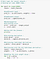Figure 113: The main logic for our case study’s training model

## i. Making predictions:

i.a. A tested person is positive for the virus.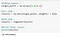Figure 114: Prediction approximate to when a tested person is positive for the virus

i.b. A tested person is negative for the virus.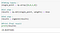Figure 115: Prediction approximate to when a tested person is negative for the virus

i.c. A tested person is positive for the virus.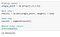Figure 116: Prediction approximate to when a tested person is positive for the virus

## j. Final weight and bias values:

In this example, we can notice that the input feature “loss of smell” influences the output the most. If it is true, then in most of the case, the person tests positive for the virus. We can also derive this conclusion from the weight values. Keep in mind that the higher the value of the weight, the more the influence on the output. The input feature “Weight loss” is not affecting the output much, so we can rule it out while we are making predictions for a larger dataset.

# Putting it all together:

`# Import required libraries :import numpy as np# Define input features :input_features = np.array([[1,0,0,1],[1,0,0,0],[0,0,1,1], [0,1,0,0],[1,1,0,0],[0,0,1,1], [0,0,0,1],[0,0,1,0]])print (input_features.shape)print (input_features)# Define target output :target_output = np.array([[1,1,0,0,1,1,0,0]])# Reshaping our target output into vector :target_output = target_output.reshape(8,1)print(target_output.shape)print (target_output)# Define weights :weights = np.array([[0.1],[0.2],[0.3],[0.4]])print(weights.shape)print (weights)# Bias weight :bias = 0.3# Learning Rate :lr = 0.05# Sigmoid function :def sigmoid(x): return 1/(1+np.exp(-x))# Derivative of sigmoid function :def sigmoid_der(x): return sigmoid(x)*(1-sigmoid(x))# Main logic for neural network :# Running our code 10000 times :for epoch in range(10000): inputs = input_features#Feedforward input : pred_in = np.dot(inputs, weights) + bias#Feedforward output : pred_out = sigmoid(pred_in)#Backpropogation  #Calculating error error = pred_out — target_output  #Going with the formula : x = error.sum() print(x)  #Calculating derivative : dcost_dpred = error dpred_dz = sigmoid_der(pred_out)  #Multiplying individual derivatives : z_delta = dcost_dpred * dpred_dz#Multiplying with the 3rd individual derivative : inputs = input_features.T weights -= lr * np.dot(inputs, z_delta)#Updating the bias weight value : for i in z_delta:  bias -= lr * i#Printing final weights: print (weights)print (“\n\n”)print (bias)#Taking inputs :single_point = np.array([1,0,0,1])#1st step :result1 = np.dot(single_point, weights) + bias#2nd step :result2 = sigmoid(result1)#Print final resultprint(result2)#Taking inputs :single_point = np.array([0,0,1,0])#1st step :result1 = np.dot(single_point, weights) + bias#2nd step :result2 = sigmoid(result1)#Print final resultprint(result2)#Taking inputs :single_point = np.array([1,0,1,0])#1st step :result1 = np.dot(single_point, weights) + bias#2nd step :result2 = sigmoid(result1)#Print final resultprint(result2)`

In the examples above, we did not use any hidden layers for calculations. Notice that in the above examples, our data were linearly separable. For instance:

We can see that the red line can separate the yellow dots (value = 1) and green dot (value = 0 ).

## Limitations of a Perceptron Model (Without Hidden Layers):

1. Single-layer perceptrons cannot classify non-linearly separable data points.

2. Complex problems that involve many parameters do not resolve with single-layer perceptrons.

However, in several cases, the data is not linearly separable. In that case, our perceptron model (without hidden layers) fails to make accurate predictions. To make accurate predictions, we need to add one or more hidden layers.
Visual representation of non-linearly separable data:

DISCLAIMER: The views expressed in this article are those of the author(s) and do not represent the views of Carnegie Mellon University, nor other companies (directly or indirectly) associated with the author(s). These writings do not intend to be final products, yet rather a reflection of current thinking, along with being a catalyst for discussion and improvement.

Published via Towards AI

# Citation

`Shukla, et al., “Neural Networks from Scratch with Python Code and Math in Detail — I”, Towards AI, 2020`

# BibTex citation:

`@article{pratik_iriondo_2020,  title={Neural Networks from Scratch with Python Code and Math in Detail — I},  url={https://towardsai.net/neural-networks-with-python},  journal={Towards AI},  publisher={Towards AI Co.},  author={Pratik, Shukla and Iriondo,  Roberto},   year={2020},  month={Jun}}`

## References:

 Biological Neuron Model, Wikipedia, https://en.wikipedia.org/wiki/Biological_neuron_model

 Logic Gate, Wikipedia, https://en.wikipedia.org/wiki/Logic_gate

## Towards AI — Multidisciplinary Science Journal

### By Towards AI — Multidisciplinary Science Journal

Towards AI publishes the best of tech, science, and engineering. Subscribe with us to receive our newsletter right on your inbox. Take a look

Written by

Written by

## Towards AI Team

#### Publishing the Best of Tech, Science, and Engineering | For Authors→ https://towardsai.net/contribute | Subscribe→ https://towardsai.net/subscribe — @Towards_AI